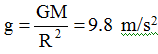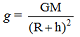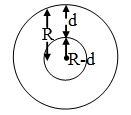Ex﻿

# 4. Gravitation

#### Variation in the value of Gravitational Acceleration (g)

(A) Variation with altitude or height :
When a body moves above the earth's surface the distance of the body from the centre of earth increases there by decreasing the force of attraction.; at the earth's surface.; at a height h above the earth's surface.
As we go above the earth's surface the value of g goes on decreasing.(B) Variation with depth d :
As we go deeper inside the earth, the body gets attracted by the core of the earth which is smaller in mass.As we go inside the earth, the value of g decreases.
Force of attraction decreases and thus decreasing the value of g and becoming zero at the centre.

(C) Variation due to rotation of the earth :
Due to the rotation of the earth, the weight of a body is maximum at the poles and minimum at the equator.

If you want to give information about online courses to other students, then share it with more and more on Facebook, Twitter, Google Plus. The more the shares will be, the more students will benefit. The share buttons are given below for your convenience.
×

#### NTSE Physics (Class X)

• Electricity
• Magnetic Effect of Curent
• Light Reflection
• Light Refraction
• Human Eye & Colourful World
• Source of Energy
• Motion

#### NTSE Physics (Class IX)

• Force & laws of motion
• Work and Energy
• Gravitation
• Sound

SHOW CHAPTERS